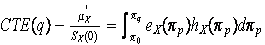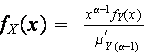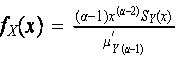Math 395 Risk Theory

Fall 2006

Instructor – James G. Bridgeman

syllabus for the course

(note use of the EXCEL functions OFFSET and SUMPRODUCT)

EXCEL Example for Panjer Recursion (try convolution on this one first!)

Some Best Fit Alternatives For 11-29 Assignment (see page 145)

last day handout part 1 Note correction in next to last line on page 5 … it’s “MX(R)-1 starts out…”

Example of Compound Geometric and Panjer Recursion For Ruin Probabilities

For much more on all of the ruin theory see the classic Gerber and Shiu “The Time Value of Ruin” Actuarial Research Clearing House (ARCH) vol. 1

and (same title) North American Actuarial Journal vol. 2 #1

Final exam will be open-book and open-notes

Cumulative Assignments  (thru 12-8)

Read Sec. 8.5 for background only

Sec. 8.1-8.4 and exerc. 8.1-8.3, 8.6-8.7, 8.9-8.18

Sec. 7.1-7.2

Study the Stop-Loss Example and Spreadsheet above … be able to do such problems independently

Sec. 6.8-6.10 (read these 3 for background only)

Sec. 6.5-6.7 and exerc. 6.29-6.35, 6.37-6.58

For the example on pages 143 to 145 (same as convolution example above) find each of the 5 two parameter distributions (normal, lognormal, inverse Gaussian, gamma, Pareto) that best fits the mean and variance of the aggregate losses.  Which of the 5 is the best choice? Hand in this work on the 29th

Sec. 6.1-6.4 and exer. 6.1-6.27

Sec. 4.6.10-4.6.11, 5.6 and exer. 4.56-4.60, 4.62,5.24-5.27

Sec. 4.6.7-4.6.9 and Exer. 4.41-4.47, 4.50-4.55

Calculate the coefficients of skewness and kurtosis for the Poisson, Neg. Binomial, and Binomial distributions

Sec. 4.6.1-4.6.6 and exer. 4.40, 4.42-4.49, and use Faa’s formula to calculate the first 4 raw and central moments of the Poisson, Neg. Binomial, and Binomial distributions

Validate (comparing formulas is good enough, but surface interpretation is interesting) that if X is a log-logistic then the k-th conditional tail moment distribution of X is a transformed beta (or, when γ=1, a generalized Pareto)

Determine the coefficient of skewness in example 5.15 (surface interpretation!)

Write down the formula for the 3rd moment analogous to Theorem 5.14 (surface interpretation!)

Sec. 5.1 – 5.5 and exer. 5.1 to 5.23 (in ch. 5 try to think in terms of the surface interpretation)

Sec. 4.5 and exer. 4.37-4.39

Exer. 4.33-4.36

Sec. 4.3-4.4 and exer. 4.13-4.32 (keep a bookmark in appendix A!)

Sec. 4.1-4.2 and exer. 4.1-4.5, 4.7-4.9, 4.11-4.12

Sec. 3.3 and exer. 3.20-3.24

Sec. 3.1-3.2 and Exer. 3.1-3.19

Ch. 2 and Exer.2.1-2.5

Project Topics: (pick any two to submit by end of semester … topics will be added as we go)

#1 Critique the “proof” given in class that vanishing of y kS(y) as y goes to infinity implies existence of the k-th moment (assume non-negative support and nice behavior for y near 0)

#2 The surface interpretation shows that the size of a stationary population is proportional to the average lifetime (expectation of life at birth).  What does the surface interpretation say about the size of a stably growing population (the rate of births at time t is Bt = B0ek t for some constant k)?

#3 Make three dimensional visual illustrations for the surface interpretation, including 2nd and 3rd moments and the relation of e(d), e2(d), and e3(d) to E[X],

E[X2], E[X3], E[X^d], E[(X^d)2], and E[(X^d)3].

#4 See handout on LX(u)=E[x^u].  Answer the series of questions.  (Think of a stationary population).

#5 Make a three dimensional visual illustration for the relationship below, and write down an interpretation in words.#6 Prove Faa’s Formula.

#7 Work out the definitions and properties of a family of severity distributions analogous to the transformed beta family, but based upon transformations of the log-Laplace distribution rather than the log-logistic.

#8 Work out the definitions and properties of a family of severity distributions analogous to the transformed beta family, but based upon transformations of the lognormal distribution rather than the log-logistic.

#9 (speculative) Work out what constraints in a maximum entropy derivation correspond to each member of the transformed gamma and transformed beta families.

#10 Work out what happens in the transformed beta and transformed gamma families if you replace the α-th conditional tail moment distributions:with the α-th equilibrium distributions):How do the resulting distributions differ from the gamma, transformed gamma, generalized Pareto, and transformed beta (that arose from the α-th conditional tail moment distributions)?

#11 Work out the definitions and properties of an inverse logistic and reciprocal inverse logistic family of distributions, analogous to the inverse Gaussian and reciprocal inverse Gaussian presented in class.

#12 Work out the definitions and properties of a family of severity distributions analogous to the transformed beta family, but based upon transformations of the inverse logistic distribution developed in project #11.

#13 Work out the definitions and properties of a family of severity distributions analogous to the transformed beta family, but based upon transformations of the true inverse Gaussian distribution presented in class rather than the log-logistic.

#14 Prove (or, if a formal proof eludes you, just illustrate and discuss the fact) that the negative binomial is like a Poisson with contagion; i.e. the negative binomial with parameters (r,β) gives the number of events in unit time if the probability of one event in infinitesimal time dt, conditional on exactly m events having occurred from time 0 to time t, is equal to dt(rβ)((1+m/r)/(1+βt)).   Try to make a similar interpretation of the binomial distribution.

#15 Show (using probability generating functions) that a mixed Poisson distribution with infinitely divisible mixing distribution is also a compound Poisson, and give two specific examples of the phenomenon.

#16 Prove the Panjer recursion formula for an (a,b,1) primary distribution using Faa’s formula.

#17 Come up with a spreadsheet (or other programming) algorithm to generate sets {j k} with ∑ (k=1, ∞) j k k = n, n=0,1, 2, … Is this efficient enough to warrant replacing Panjer recursion with direct use of Faa’s formula to calculate compound distribution probabilities for (a, b, 0) primary distributions?  Note that this would give you a calculation technique anytime the probability generating function of the primary distribution is known, whether or not there is a recursive feature to it.  Is this an improvement versus brute force convolution?

#18 Develop an approximation formula (based on the surface interpretation) for E[(S-(j+1)·h)+k] in terms of E[(S-j·h)+k] for k=2 and 3 that would apply in general, whether or not S has been discretized.  Write down the more simplified recursion formula this gives when S has been discretized.

#19 Make up examples using both a compound Poisson and a compound Negative Binomial process (and pick one or more severity distributions to give yourself examples) and study how much, if any, difference there is in your approximation for the upper limit exp(-R~u) on the discretely observed ruin probability ψ~(u) if you use a third order approximation for the adjustment coefficient R~ rather than the second order approximation that is usually used.  In other words, quantify the contribution of skewness in the severity to an estimate for the upper limit of the ruin probability.

#20 Develop the logic and formulas for a compound negative binomial process in the continuously observed collective risk ruin model.  Point out the most significant departures from the conclusions of the corresponding compound Poisson process.